# Warping Text to a Bézier curves

May 29, 2009

## Background

A while back I got curious about how certain text effects could be achieved, and one of the things I explored was warping text along a curve to achieve a kind of sweeping effect. I created a prototype for this, to explore different solutions. This article contains some general notes on the subject, as I reflect back on this work. The information given here could be used as a kind of cookbook to replicate the work. The original work was done using C# and GDI+, although the concepts are applicable to other frameworks.

## Bendy Text

Given some arbitrary spline, how can text be drawn in such a way that it appears to follow the curve in a way that is appealing to the eye?A sample of the desired result.

Looking around the interwebbietubes, there seemed to be few examples of doing this, and most examples were crude, i.e. involving rotating individual letters with linear transforms to align each character with the curve, which results in a very odd, non-fluid appearance. Much good information was gleaned from old articles written by Don Lancaster, in particular one about Nonlinear Graphics Transforms from 1995. As always, it is good to keep in mind that in this field, we stand on the shoulders of giants. Many thanks to Don for putting so much good and accessible information out there over the years.

## Vector text

Normally when thinking about drawing text in GDI+, most people think of Graphics.DrawString() and the like, which given a string will draw into a Graphics context. The result is a bunch of pixels, not exactly useful for doing non-linear transformations. What would be preferable is to get vector outlines of the rendered text, with points that can be transformed to get the desired effect. Fortunately, GraphicsPath provides a mechanism for doing this, even if the API is a little obtuse. It helps to understand what a GraphicsPath is: GraphicsPath is a form of display list, consisting of a very small number of simple vector primitives. Pixels just don't exist as far as a GraphicsPath is concerned, until it's rendered using Pens and/or Brushes. In fact, here is a complete list of all the primitives that are used under the hood by GraphicsPath:

• Start: Defines a point that is used to denote the starting point of a path
• Line: a line. includes the endpoint, the start point was the last point from the last instruction.
• Bezier: a cubic Bézier curve. Defines 3 of the 4 control points, the start point is the last point from the last instruction
• Bezier3: A quadratic Bézier curve. (see below)
• CloseSubpath: marks the endpoint of a subpath this is used for
• PathMarker: defines a marker for a path. Not used for rendering as far as I can tell, this is for information purposes
• DashMode: marks a segment as dashed, which gives a suggestion on how it should be rendered

There are just two that render something visible when a GraphicsPath is drawn: Line and Bézier. Note that the MSDN documentation mixes up quadratic and cubic Splines. (Imagine that! misleading MSDN docs. Grumble.) In practice, I've never run across a GraphicsPath that actually contains a quadratic spline (Bezier3).

What about other shapes?   After all, an ellipse can be added to a GraphicsPath, it must have an ellipse primitive, right? Nope. If an ellipse is added to a GraphicsPath, under the hood it will be approximated using several Bézier splines.

What about adding text to a GraphicsPath? Once again, it reduces to a bunch of points and instructions for lines and cubic Béziers. These are derived directly from the description of the font, which is vector based as well. The nice thing is that if a bunch of things drawn into a GraphicsPath, one can iterate over these primitives and get a complete description of what it is doing under the hood. And with that information, an identical path can reconstructed from the primitives. During the process, the points can be pushed around using some kind of transform to achieve the text warping effect.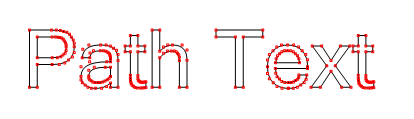Text added to a GraphicsPath then rendered without fill.
Then all points from the path are marked with red (including control points for splines)

## Bézier curves

For my first pass at this, I decided to use a Bézier spline to define the path of the text. Cubic Béziers are supported by GDI+, there are methods for drawing them on the Graphics object, and they are one of the few primitives supported by GraphicsPath. The formulas for Béziers are simple to calculate, they can be used to approximate other curves, and getting a set of points along a cubic Bézier given its four control points is mostly a trivial exercise. More complex shapes/curves would come later.

Getting a point on a Bézier curve is not something that GDI+ provides for, so understanding the formulas for cubic Béziers is necessary for this work. I won't go into exhaustive detail on the math behind this, as plenty of information is readily available on this subject, from Wikipedia and elsewhere.

## Formulas for cubic Béziers

OK, math time! It is most illustrative to look at how a cubic Bézier is constructed geometrically, which is pictured below.The geometric construction of a cubic Bézier (image from Wikipedia)

The curve is defined by four control points P0 through P3. The first and last point are the start and end point of the curve, the other two are colloquially referred to as "handles" to control the shape of the curve. The four control points can be connected to form three line segments (pictured in gray). Three points are interpolated using the parameter t on the line segments connecting the control points, and these form the two green segments above. A final line segment is constructed by interpolating points along the previous two segments for t in the same way (shown in blue). A final point is interpolated along this last line segment, and that point is a point on the curve for value t.

The linear interpolation (lerp) between two scalar values is defined as follows:

````vlerp = v0 + ( v1 - v0 ) * t `
```

From which one can create a lerp function between two 2D points:

``````lerp(P0, P1, t) :
xlerp = x0 + ( x1 - x0 ) * t
ylerp = y0 + ( y1 - y0 ) * t
return point (xlerp, ylerp)```
```

The geometric picture above can then be expressed as follows:

``````P4 = lerp(P0, P1, t);
P5 = lerp(P1, P2, t);
P6 = lerp(P2, P3, t);
P7 = lerp(P4, P5, t);
P8 = lerp(P5, P6, t);
P9 = lerp(P7, P8, t);

Where P9 is the point on the curve for t.```
```

A cubic Bézier can also be expressed as a cubic polynomial with eight coefficients: (I'll describe how to calculate the coefficients from the four control points in a later)

``````x = At3 + Bt2 + Ct + D
y = Et3 + Ft2 + Gt + H ```
```

For values of t between 0 to 1, these polynomials produce the x and y coordinates for a point on the curve. Values outside of that range continue the function out to infinity somewhere. The formula is really the same for the x and y versions, just with different coefficients. The equations independently relate x and y to the value parametric value t. Because of this splines can be extended into three dimensions (or more!) very easily.

So where do those coefficients (A..H) come from? For a full explanation of the math, check the resources section at the bottom, but for now I'll just give the formulas for converting control points to coefficients.
Given four control points for the spline P0 .. P3, having values of (x0,y0) .. (x3,y3) , the coefficients are:

``````A = x3 - 3 * x2 + 3 * x1 - x0
B = 3 * x2 - 6 * x1 + 3 * x0
C = 3 * x1 - 3 * x0
D = x0

E = y3 - 3 * y2 + 3 * y1 - y0
F = 3 * y2 - 6 * y1 + 3 * y0
G = 3 * y1 - 3 * y0
H = y0```
```

Should it be necessary to calculate control points from coefficients, here are the inverse operations:

``````x0 = D;
x1 = D + C / 3
x2 = D + 2 * C / 3 + B / 3
x3 = D + C + B + A

y0 = H;
y1 = H + G / 3
y2 = H + 2 * G / 3 + F / 3
y3 = H + G + F + E```
```

So to illustrate what has been covered so far, here is some pseudocode, using control points P0..P3:

``````// draw the Bézier using GDI+ function (g is Graphics object)
g.DrawBezier(Pens.Black,P0, P1, P2, P3);

// draw lines connecting the control points

g.DrawLine(redPenWithEndCap, P0,P1);
g.DrawLine(redPenWithEndCap, P2,P3);

[[compute coefficients A thru H as described above]]

// draw 20 points, with a fixed increment for the parameter t
for (float t = 0; t <= 1; t += 0.05f)
{
x = At3 + Bt2 + Ct + D
y = Et3 + Ft2 + Gt + H

// call function that draws a filled rect at x,y coord.
DrawBoxAtPoint(g, Color.Blue, x, y);
}
``````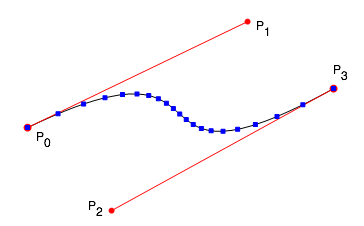Pseudocode output. GDI+ spline is in black.
Control points are connected with red lines.
The 20 points calculated are marked in blue.

As t varies from 0 to 1, these points "travel" along the curve from the start point to the end point. If enough values are used, a good approximation of the Bézier could be drawn. In fact, under the hood, most graphics systems draw Bézier curves using recursive subdivision, dividing the curve to the level of pixels or sufficiently small enough to draw with short line segments.

One other interesting thing to note is that even though the values for t used varied by a fixed increment, some of the resulting points are closer together than others, depending on where they lie on the curve. Any two sequential points may have different "arc-lengths" (distance measured along the curve, rather than straight-line distance) from another two. It may help to think of it as the rate at which the output values move along the curve "speeds up" and "slows down" even though the change in t is constant. We'll revisit to this later.

## Tangents and perpendiculars

In order to use the text points with the Bézier formula, the x values must first be normalized (scaled) into the range of 0..1, so that they used for the t parameter of the Bézier formula. If the text starts at or near the X origin, and the width of the text is known, this normalization is done simply by:

````xnorm =  x / textwidth`
```

The Y coordinates for the text need to project out away from the curve in a direction perpendicular to the curve at that point. In order to do that, a vector is needed that is perpendicular to the curve for the point produced by t. That can be obtained by finding the tangent for the curve at that point, and rotating it 90 degrees.

Calculating the tangent vector for a Bézier is trivial, as it is simply the derivative of the Bézier polynomial:

``````Vx = 3At2 + 2Bt + C
Vy = 3Et2 + 2Ft + G ```
```

We can rotate the vector 90 degrees by using linear algebra, or by simply swapping the terms and negating one of them. If V = (x, y) then Vrotate90 = (y, -x) or (-y, x), depending on which way one wants to rotate the vector. That gives a vector that is perpendicular to the Bézier for the point produced by the parameter t. Drawing those perpendicular vectors on top of the points would look something like this: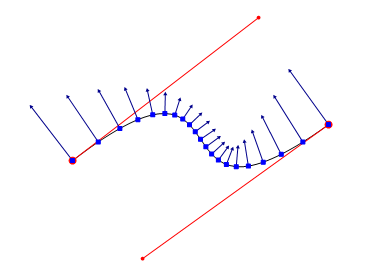Raw Perpendicular vectors. These have been shortened for illustrative purposes.
(those are supposed to be little arrows on the ends)

The Y coordinates for the text points need to be translated in the direction defined by the perpendicular vectors, but the magnitude (length) of those vectors is not important, just their direction. For convenience the perpendicular vectors can to be normalized into unit vectors, making them all exactly 1 unit long. For a vector having components x and y, this is done as follows:

``````magnitude = sqrt( x2 + y2 )  // distance formula
x = x / magnitude
y = y / magnitude
// note, special case: if magnitude is 0, x,y is either 0 or undefined```
```Normalized perpendicular vectors, for 20 points defined by parameter t.
Vectors drawn with lines 10 pixels long for illustrative purposes.

That covers most of the math needed to make a first pass at warping text onto a Bézier curve.

## First attempt: Stretchy Text

Here is a first try at warping text, given Bézier control points P0..P3, and a graphics context g to draw on:

``````string text = "Some text to wrap";

[[ Calculate coefficients A thru H from the control points ]]

GraphicsPath textPath = new GraphicsPath();

// the baseline should start at 0,0, so the next line is not quite correct
path.AddString(text, someFont, someStyle, someFontSize, new Point(0,0));

RectangleF textBounds = textPath.GetBounds();

for (int i =0; i < textPath.PathPoints.Length; i++)
{
PointF pt = textPath.PathPoints[i];
float textX = pt.X;
float textY = pt.Y;

// normalize the x coordinate into the parameterized value
// with a domain between 0 and 1.
float t =  textX / textBounds.Width;

// calculate spline point for parameter t
float Sx = At3 + Bt2 + Ct + D
float Sy = Et3 + Ft2 + Gt + H

// calculate the tangent vector for the point
float Tx = 3At2 + 2Bt + C
float Ty = 3Et2 + 2Ft + G
// rotate 90 or 270 degrees to make it a perpendicular
float Px =   Ty
float Py = - Tx

// normalize the perpendicular into a unit vector
float magnitude = sqrt(Px2 + Py2)
Px = Px / magnitude
Py = Py / magnitude

// assume that input text point y coord is the "height" or
// distance from the spline.  Multiply the perpendicular vector
// with y. it becomes the new magnitude of the vector.
Px *= textY;
Py *= textY;

// translate the spline point using the resultant vector
float finalX = Px + Sx
float finalY = Py + Sy

// I wish it were this easy, actually need
// to create a new path.
textPath.PathPoints[i] = new PointF(finalX, finalY);
}

// draw the transformed text path
g.DrawPath(Pens.Black, textPath);

// draw the Bézier for reference
g.DrawBezier(Pens.Black, P0,  P1,  P2,  P3);```
```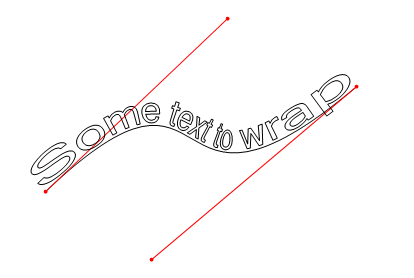Output of pseudocode, with control points added

Looks pretty good, but there are a few problems. The text appears scrunched together in the middle, and stretched out on the ends. Remember that the arc-length between points can vary, even if t is incremented by a fixed amount. I'll add some points using an fixed increment for t, and overlay direction vectors to show how they were used to warp the text: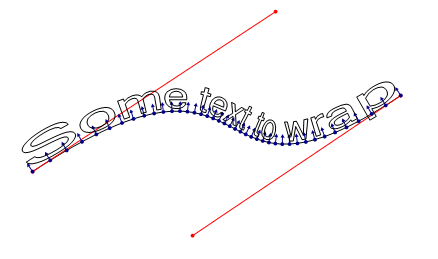Illustrating that the formula for a Bézier is not
arc-length parameterized, and the problem this creates.

As the parameter t varies by a fixed amount, the output points have varying arc-lengths from one to the next. This characteristic of the Bézier formula is what causes the text to be compressed or stretched along the curve.

There is another problem with the algorithm: text is always stretched or compressed to fit the length of the curve, as shown below:Text squashed to fit because of directly mapping its width to the domain of t (0..1)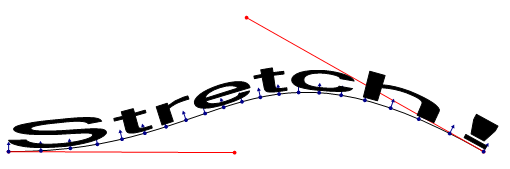Text stretched to fit because of directly mapping its width to the domain of t (0..1)

What would be better is to map the text's x coordinate to the parameter t in such a way that the text does not try to exactly fit the text to the full length of the Bézier. In order to do that, the arc-length of the curve must be known. This problem is related to the first one.

## Fixing the text length problem (and how long is that Bézier, anyway)

The fix to the second problem (total text length) is trivial, if the arc-length of the curve is known. Rather than map the actual width of the text from 0..1 for t, the text can be drawn into a bounding box that is the same width as the arc-length of the spline, then x coordinates of the box are scaled to 0..1 by dividing all x coordinates by the arc-length. This is a little easier to understand visually:Bézier above has an arc-length of 500 units, so a 500 unit wide bounding box
(shown at top in blue) is used for text, and scaled to the domain of 0..1.
Bounding box is shown as it would look if it were somehow wrapped to the curve.

The text can also be clipped to the bounding box to prevent it from overrunning the end of the spline.

That leaves the problem of calculating the arc-length of a cubic Bézier curve. After doing some research, I discovered that there is apparently no closed form solution to this problem. There are a number of ways of approximating the arc-length, some involving moderately complex calculus. Even the most sophisticated and accurate solutions involve an iterative approach. (See resources for more info).

However, there is a very simple way to estimate the arc-length: divide the curve into a bunch of line segments based on a fixed increment for t, then sum the straight-line length of those line segments. It turns out that even a small number of divisions can give a fairly good estimate, as it exploits a characteristic of the Bézier formula that the output points are close together in sharp curves. Some empirical investigation showed that around 100 divisions would yield estimates with less than .001 error, which would be half a pixel for a 500 pixel long curve. Close enough for the purpose at hand.

The process can be visualized by drawing the line segments on top of a Bézier:Estimating arc-length with four divisions.
segments shown in blue have large error.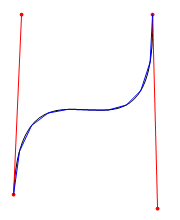With eight divisions, segments start to converge
on the curve, estimation error is smaller.

The pseudocode for this is very simple. There are a few optimizations that can be made, but the basic algorithm is fairly efficient as long as the number of divisions is not too large.

``````int numberOfDivisions = 100;
int maxPoint = numberOfDivisions + 1;

// _formula.GetPoint(t) is assumed to take a parameter t and return
// a point using the Bézier formula.  The _formula object would
// have the Bézier coefficients to calculate this.

PointF previousPoint = _formula.GetPoint(0);  // for Bézier == P0 control point

float sum = 0;

for (int i = 1; i <= maxPoint; i++)
{
PointF p = _formula.GetPoint( i / (float) maxPoint );
sum += distance(previousPoint, p);
previousPoint = p;
}

// function to calculate straight-line distance between two points:
float distance(PointF a, PointF b) { return sqrt( (bx - ax)2 + (by - ay)2 ); }```
```

This code could easily be generalized for any single-valued parameterized algorithm that is continuous, has a fixed domain, and returns points, such as the formula for an ellipse or different types of splines.

It may be possible to estimate the error and dynamically pick an "ideal" number of divisions. This could be done by subdividing until segment lengths are below some threshold or by comparing the tangents of the line segments to the tangents of the curve, but I have not explored either of these ideas. Fixing the number of divisions at around 100 seems to work well enough for the purpose at hand.

## Arc-length parameterization

Even after the text width problem is fixed, there is still an issue with text getting squashed or stretched due to the non-uniform arc-lengths between uniform values of t.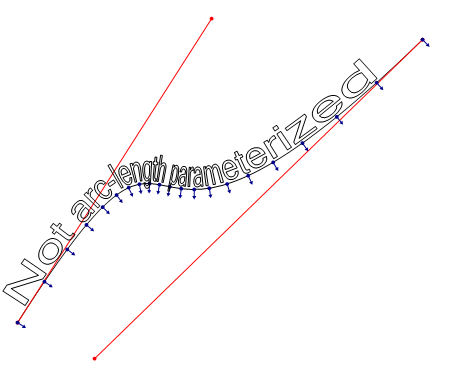This graphic illustrates that the lack of arc-length parameterization.
This issue is independent of the other problem, but has a related solution.
(Perpendicular vectors rotated 180° so they are not obscured)

The solution to this problem is called arc-length parameterization. The idea is to map an input value u to a value t which can be passed to the Bézier formula, and will produce points that have uniform arc-length distances for uniform values of u. Stated another way, for values 0..1 for u it would map to values of t in such a way to produce a point that is a fractional arc-length distance from the start of the curve equal to u. For example, a value of .25 for u would always produce a point that is one-fourth of the arc-length distance along the curve. The intermediate value of t in this case is unknown, and must be calculated by the mapping function somehow.

The implementation I came up with (based on suggestions from friend who is much more mathematically inclined than I) was to approximate this mapping function using a table of arc-lengths. It consists of saving a list of the sums produced by the arc-length algorithm already covered. For a spline having an arc-length of 500, divided into 100 divisions, the table might for a certain spline might look like this:

```  0.0
5.2
10.5
15.7
... (more points) ...
494.8
500.0
```

The index of values in this table equates to some parameter t, for example, if the list contained 101 values, index 0 contains the arc-length for t=0.0, index 100 has the arc-length for t=1.0, and index 50 contains the arc-length for t=.5. If the table were stored in a 0-indexed array called arcLengths, the value of t for a given index would be:

````t = index / (float) (arcLengths.Length - 1)`
```

Given such a table, it is possible to find values of t that are close to a desired arc-length. So we could take and input value of u, calculate the desired arcLength (u * totalArcLength), then find the index of the largest entry in the table that is smaller than or equal to the desired arcLength. This could be done using a brute force traversal, or preferably, a binary search. If this value happens to be equal to the arcLength, then the value of t for the input value of u can be calculated as previously described. More often then not, the search will find the largest value that is smaller than the desired arc-length. In this case, linear interpolation can be used between the found entry and the next one to find an estimate for t. Assuming sufficient divisions were used to create the table, the distance between those to points will be sufficiently small that the error for the mapping will be negligible.

The following pseudocode demonstrates the technique:

``````
float u = // the parameterized value, assumed to be between 0 and 1
float t; // we are attempting to find t for u

float [] _arcLengths = // a pre-calculated list of arc-lengths along the Bézier

// get the target arcLength for curve for parameter u
float targetArcLength = u * _arcLengths[ _arcLengths.Length - 1 ];

// the next function would be a binary search, for efficiency
int index = IndexOfLargestValueSmallerThan(_arcLengths, targetArcLength)

// if exact match, return t based on exact index
if (_arcLengths[index] == targetArcLength)
{
t = index / (float) (arcLengths.Length - 1);
}
else  // need to interpolate between two points
{
float lengthBefore = _arcLengths[index];
float lengthAfter = _arcLengths[index+1];
float segmentLength = lengthAfter - lengthBefore;

// determine where we are between the 'before' and 'after' points.
float segmentFraction = (targetLength - lengthBefore) / segmentLength;

// add that fractional amount to t
t = (index + segmentFraction) / (float) (_arcLengths.Length -1);
}```
```

The basic algorithm can be generalized to arc-length parameterize the same class of functions that can be handled by the arc-length estimation code. The output, when using this mapping algorithm, is much more appealing: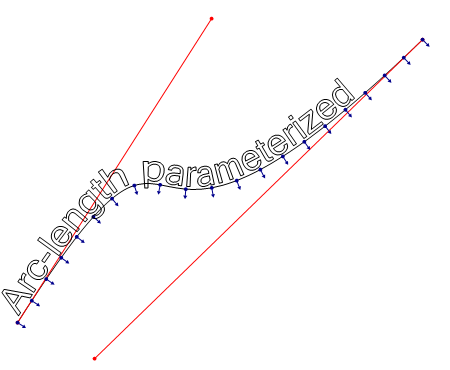After arc-length parameterization. Note the uniform values
for u (plotted in blue) are now evenly distributed on the curve.
(Perpendicular vectors shown rotated 180° so they are not obscured)

## Solving other minor issues

The algorithms presented still may have some minor issues. In particular, long horizontal line segments may cause the characters to distort when going around a sharp curve. This problem can be overcome in all but the most severe cases by iterating through the text path and dividing long line segments into shorter ones.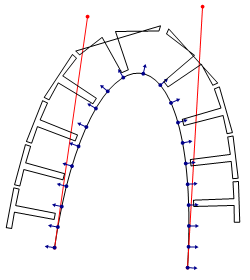The T's have a single line for the top, so it can't
bend, causing gross distortions in sharp curves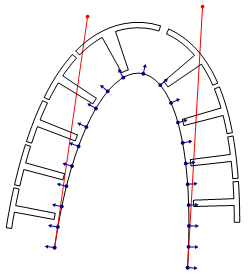Dividing long lines into sufficiently
shorter segments can improve appearance,
at some expense to performance.

In some cases, this technique can benefit from using short Bézier curves instead of short line segments. However, it is not enough to simply replace all line segments with Bézier curves, as this gives a different and sometimes unpleasant appearance for long segments. It is left as an exercise to the reader to find out why.

## Extending the techniques to complex paths

The techniques can easily be expanded to allow for warping to arbitrary paths of increasing complexity. All that need be done is calculate the arc-length of the entire path (by summing the arc-lengths of its components), and then parameterize the whole thing. A wrapper class for the formula can be written which contains multiple sub-paths as children, and could decide which child to use for a particular parameter through reverse interpolation over the total length. Alternatively this could be built into the arc-length estimation algorithm.

One could even wrap text around other text!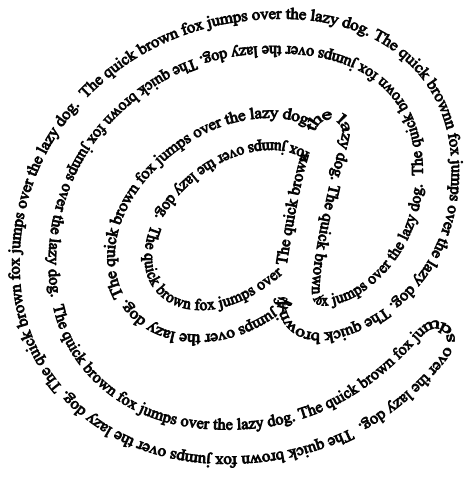Wrapping text around an arbitrary path.

This potentially introduces new problems to solve, particularly for warping around other text paths. Discontinuities (places where the path stops at one place and restarts elsewhere), can cause severe 'garbling' if a character or other object straddles those points. A good general algorithm would need to account for these, and make sure that they are handled appropriately, perhaps by pushing the characters beyond the break. Also, many characters contain acute bends and corners that may result in excessive warping that looks unpleasant (as seen in a few places in the figure above).

## Conclusion

The use of these techniques for distorting text (or any path!) can give a fairly natural and fluid appearance. The algorithms presented allow for real time manipulation of the curves, even on low-end modern hardware. Several optimizations can be made that were not covered in this article for the sake of simplicity. The techniques can be readily adapted to any graphics framework that has a vector path object (such as WPF, Cocoa, Postscript, etc). Subtle use of warped vector paths can be used for fun aesthetic effects.